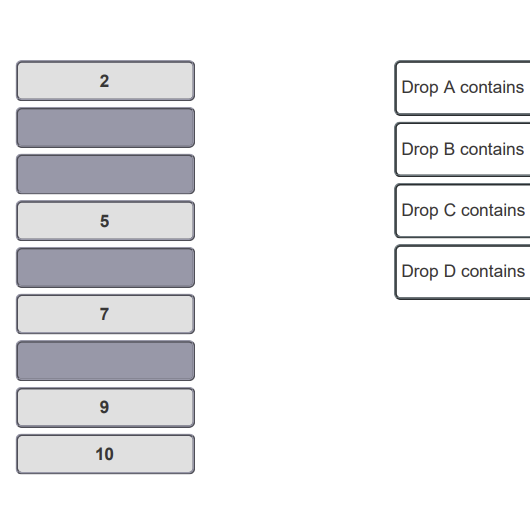# Problem: Imagine a unit of charge called the zorg. A chemist performs the oil drop experiment and measures the charge of each drop in zorgs.You may want to reference (Pages 44 - 74) Chapter 2 while completing this problem.Based on the charge of the electron you calculated from the data in Part A, complete the statements below regarding the number of electrons in each drop.

###### FREE Expert Solution

From Part A, charge of one electron is -1.4 x 10-9

Use formula:

79% (253 ratings)###### Problem Details

Imagine a unit of charge called the zorg. A chemist performs the oil drop experiment and measures the charge of each drop in zorgs.

You may want to reference (Pages 44 - 74) Chapter 2 while completing this problem.Based on the charge of the electron you calculated from the data in Part A, complete the statements below regarding the number of electrons in each drop.